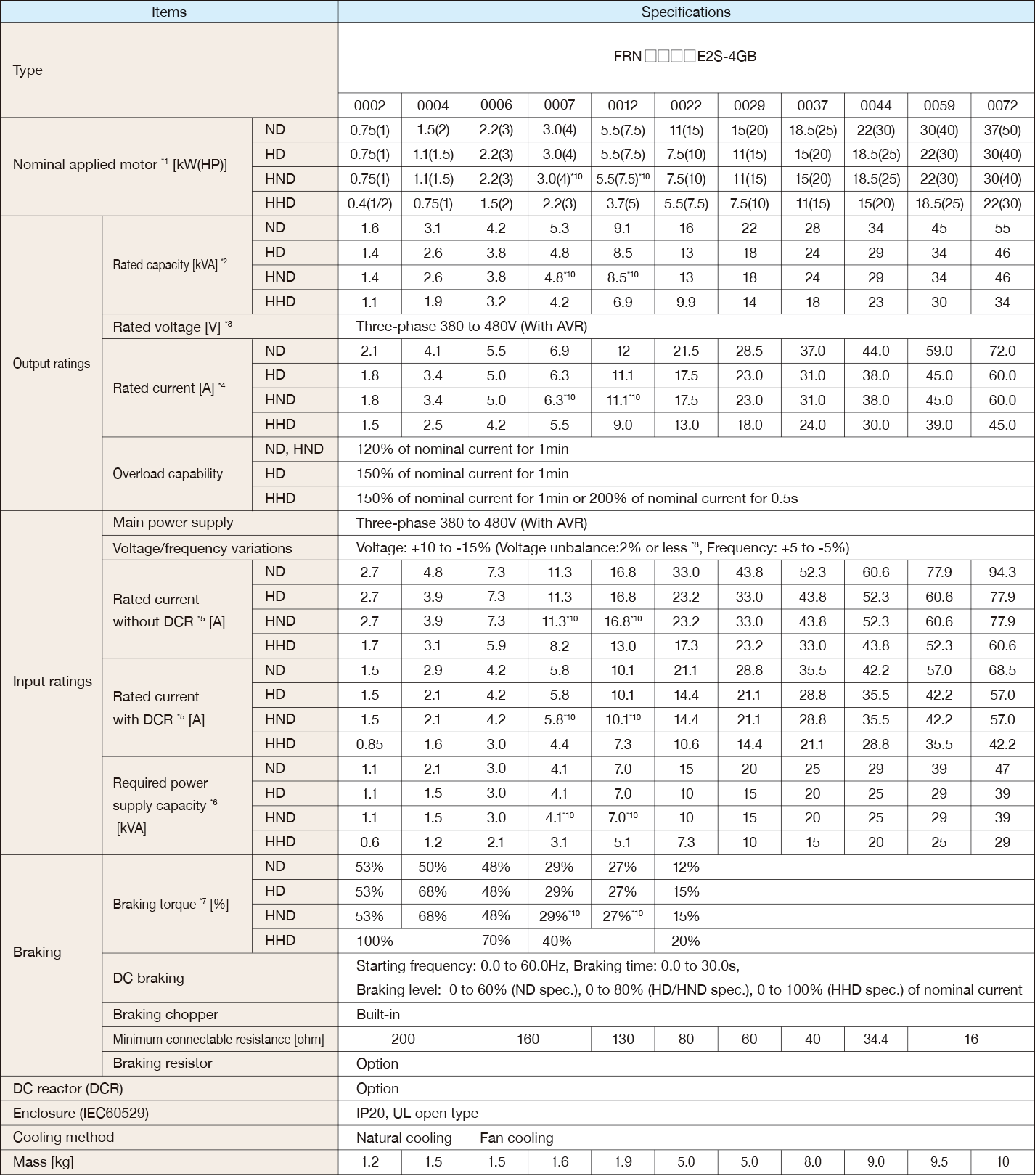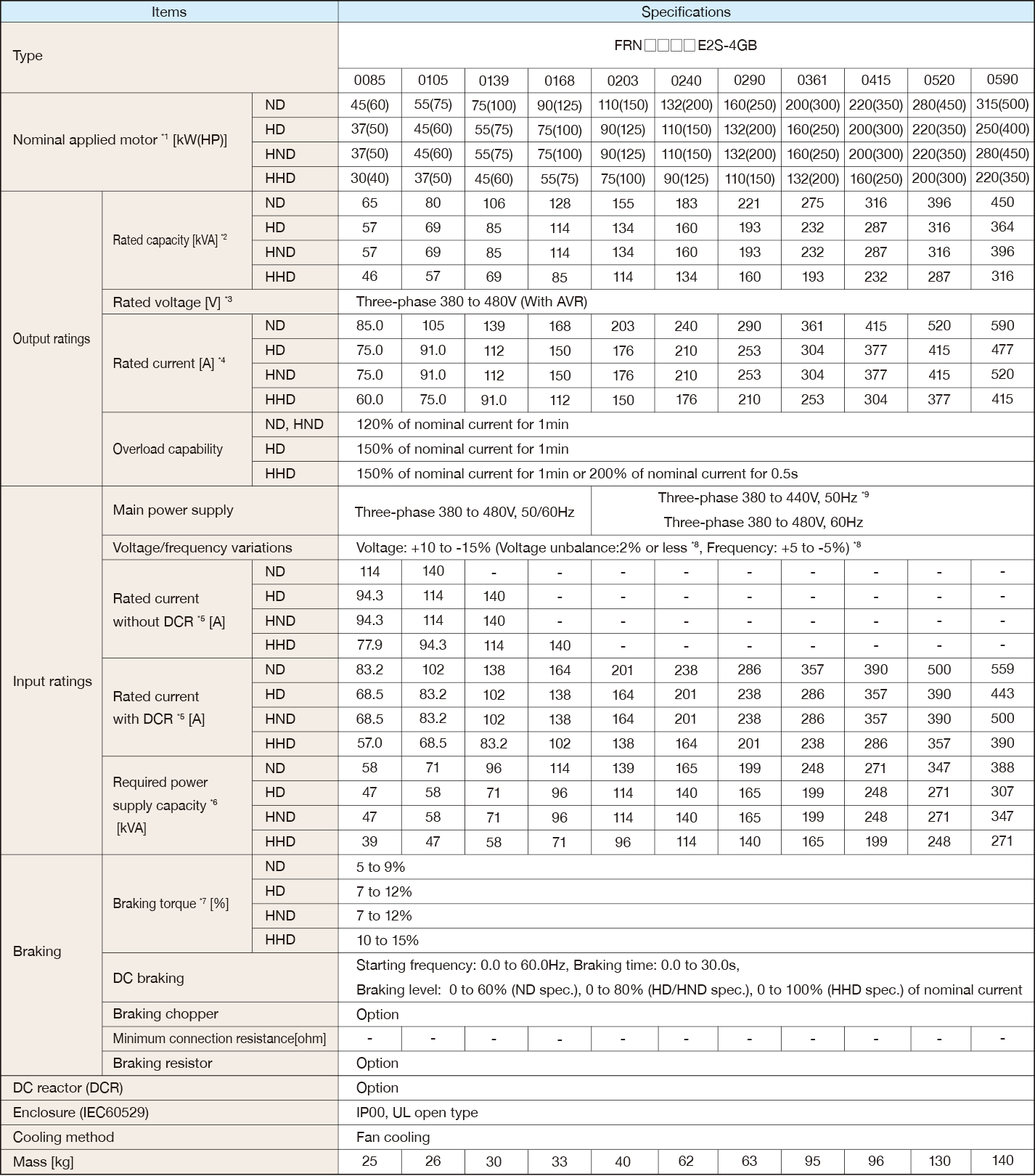## UtilitiesFrenic-Ace

High Performance Inverter

The standard inverter for the next generation, the FRENIC-Ace, can be used in most types of application- from fans and pumps to specialized machinery

Specifications

### Three phase 400V class series1* Fuji 4-pole standard motor. At the selection of the inverter rating, consider not only the rating capacity(kW) is enough but also inverter output current is larger than selected the motor's nominal current.

2* Rated capacity is calculated by assuming the output rated voltage as 440 V.

3* The output voltage cannot exceed the power supply voltage.
4* When the carrier frequency (F26) is set to below value or higher, the inverter is sure to be necessary to derate their nominal current.
HHD spec.---type 0002 to 0012 : 8kHz, type 0022 to 0168 : 10kHz, type 0203 to 0590 : 6kHz
HND spec.---type 0002 to 0012 : 8kHz, type 0022 to 0059 : 10kHz, type 0072 to 0168 : 6kHz, type 0203 to 0590 : 4kHz
HD,ND spec.---All type : 4kHz
The rated output current at HD/ND spec. is decreased 2% for every 1 °C (1.8 °F) when ambient temperature is +40 °C (+104 °F) or more.

5* The value is calculated assuming that the inverter is connected with a power supply with the capacity of 500 kVA
(or 10 times the inverter capacity if the inverter capacity exceeds 50 kVA) and %X is 5%.
Be sure to use the DCR when applicable motor capacity is 75kW or above.
*6 Obtained when a DC reactor (DCR) is used.

*7 Average braking torque for the motor running alone. (It varies with the efficiency of the motor.)

*8 Voltage unbalance (%) =(Max. voltage (V) - Min. voltage (V))/Three -phase average voltage (V) × 67 (IEC 61800 - 3) If this value is 2 to 3%, use an optional AC reactor (ACR).

*10 HND spec. of the type 0007 and 0012: allowable ambient temperature 40 °C (+104 °F) or less.
The rated output current at HND spec. is decreased 1% for every 1 °C (1.8 °F) when ambient temperature is +40 °C (+104 °F) or more.1* Fuji 4-pole standard motor. At the selection of the inverter rating, consider not only the rating capacity(kW) is enough but also inverter output current is larger than selected the motor's nominal current.

2* Rated capacity is calculated by assuming the output rated voltage as 440 V.

3* Output voltage cannot exceed the power supply voltage.

4* When the carrier frequency (F26) is set to below value or higher, the inverter is sure to be necessary to derate their nominal current.
HHD spec.---type 0002 to 0012 : 8kHz, type 0022 to 0168 : 10kHz, type 0203 to 0590 : 6kHz
HND spec.---type 0002 to 0012 : 8kHz, type 0022 to 0059 : 10kHz, type 0072 to 0168 : 6kHz, type 0203 to 0590 : 4kHz
HD,ND spec.---All type : 4kHz
The rated output current at HD/ND spec. is decreased 2% for every 1 °C (1.8 °F) when ambient temperature is +40 °C (+104 °F) or more.

5* The value is calculated assuming that the inverter is connected with a power supply with the capacity of 500 kVA (or 10 times the inverter capacity if the inverter capacity exceeds 50 kVA) and %X is 5%.
Be sure to use the DCR when applicable motor capacity is 75kW or above.

6* Obtained when a DC reactor (DCR) is used.

7* Average braking torque for the motor running alone. (It varies with the efficiency of the motor.)

8* Voltage unbalance (%) =(Max. voltage (V) - Min. voltage (V))/Three -phase average voltage (V) × 67 (IEC 61800 - 3) If this value is 2 to 3%, use an optional AC reactor (ACR).

9* The 400 V class series with type 0203 or above is equipped with a set of switching connectors (male) which should be configured according to the power source voltage and frequence

*Source: https://www.fujielectric.com/products/ac_drives_lv/frenic-ace/spec/